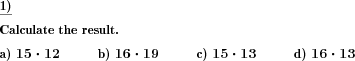Custom math worksheets at your fingertips# Details for problem "Multiplication of two natural numbers"

Quickname: 8228

Elementary School, Primary School, Junior High School, Middle School, High School, Senior High School.

## Summary

Multiplication problems with defined number range

## Example## Description

Simple multiplication problems with two factors are presented here. The factors are natural positive numbers.

The number range can be set for the first and the second factor. The number range from which the result, the product, will then come depends on the selected number ranges for the individual factors.

No particular way of solution is offered or demanded. Only the corresponding product is given as an answer in each case. Thus the task is suitable for being solved with different methods, also with a calculator.

The number of tasks is selectable.

Download free printable worksheets for this math problem here. The worksheet contains the problems only, the solution sheet includes the answers. Just click on the respective link.

•Worksheet 1Solution sheet with answers
•Worksheet 2Solution sheet with answers
•Worksheet 3Solution sheet with answers

If you can not see the solution sheets for download, they may be filtered out by an ad blocker that you may have installed. If this is the case, please allow ads for this page and reload the page. The solution sheets will then reappear.

• Do these sample worksheets do not really fit?
• Do you need more math worksheets, with a different level of difficulty?
• Would you like to combine different problems on a worksheet and adjust them to your needs?
• As a teacher, you can put together your own worksheets using the automatically generated math problems provided.
With a free initial credit, you can start creating your own math worksheets in a few minutes.

You can try it for free! Register here, to create custom worksheets now!

## Customization options for this problem

Parameter
Possible values
Number of problems
1, 2, 3, 4, 5, 6, 7, 8, 9, 10
First factor range
9, 19, 49, 99, 199, 499, 999, 9999, 99999
Second factor range
9, 19, 49, 99, 199, 499, 999, 9999, 99999

## Similar problems

Remark
Description
As a "double the number" problem
Double a number with or without decimal places.
Problems with solution in long multiplication form
Two factors have to be multiplied using the long multiplication written form.
Is also available as a variant with powers of ten
Multiplication of a whole numbers with powers of ten or multiples of powers of ten
Multiplication of negative numbers
Multiplication of two negative numbers, training the rules for the product's sign.
Preparation for long multiplication: multiply via decomposition of factor
Preparation for long multiplication: Multiply by decomposing first factorDeutsche Version dieser Aufgabe
These informational pages with samples describe math problems that can be combined on custom math worksheets with solutions for home and K-12 school use.
Deutsche Seiten
×